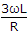# Electronics and Communication Engineering - Signals and Systems - Discussion

5.

In an ac circuit the fundamental component of current wave lags the corresponding voltage wave by 20°. The third harmonic component of current wave lags the corresponding voltage by an angle.

 [A]. less than 20° [B]. more than 20° [C]. equal to 20° [D]. equal to or more than 20°

Explanation:

For third harmonic θ = tan-1 =.

 Engrafridi said: (Nov 18, 2015) How can any solve it?

 Chaitu said: (Jan 5, 2017) How to solve this?

 Dado said: (Mar 25, 2017) tan(θ)=n* first harmonic. θ = tan^-1(3 * wL/R).

 Dhinesh Kumar said: (Sep 2, 2017) How to get the WL/R?

 Np Naresh said: (Sep 17, 2017) Explain briefly.

 Ganesh said: (Oct 18, 2017) How to solve this one?

 Saira Narasimha Reddy said: (Dec 30, 2019) Theta = tan inverse (XL/R)= 20° When, 3rd hormonic theta = tan inverse (3XL/R) so, Value of theta increased more than previous so, More than angle 20°.